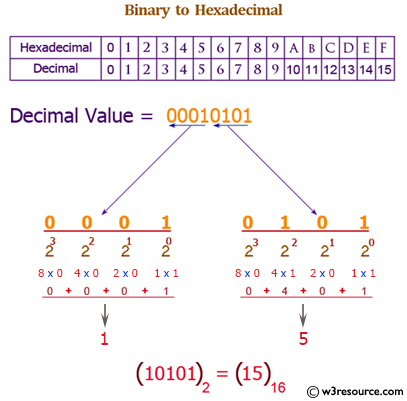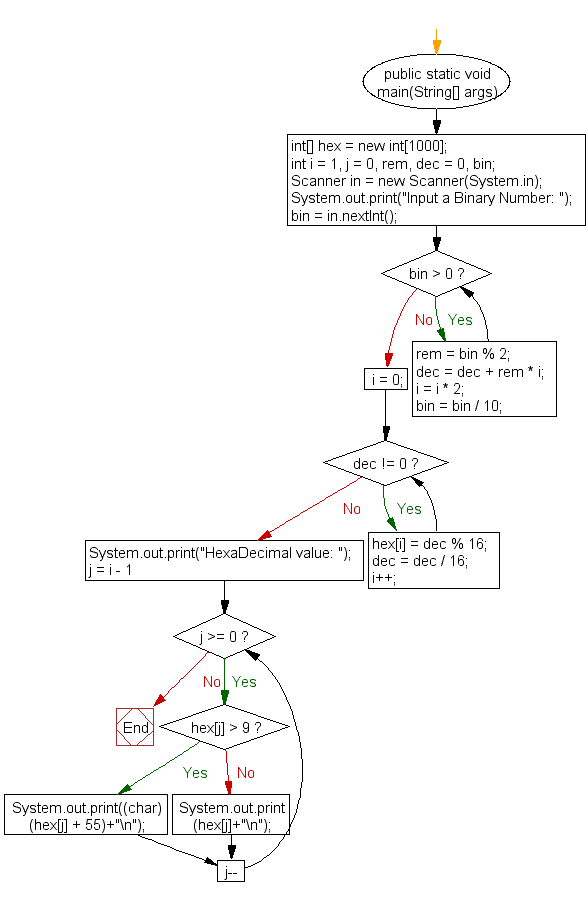﻿ Java exercises: Convert a binary number to hexadecimal number - w3resource# Java Exercises: Convert a binary number to hexadecimal number

## Java Basic: Exercise-23 with Solution

Write a Java program to convert a binary number to hexadecimal number.

Binary number: A binary number is a number expressed in the base-2 numeral system or binary numeral system. This system uses only two symbols: typically 0(zero) and 1(one).

Hexadecimal number: This is a positional numeral system with a radix, or base, of 16. Hexadecimal uses sixteen distinct symbols, most often the symbols 0-9 to represent values zero to nine, and A, B, C, D, E, F (or alternatively a, b, c, d, e, f) to represent values ten to fifteen.

Test Data:
Input a binary number: 1101

Pictorial Presentation: Binary to Hexadecimal numberSample Solution:

Java Code:

``````import java.util.Scanner;
public class Exercise23 {
public static void main(String[] args)
{
int[] hex = new int;
int i = 1, j = 0, rem, dec = 0, bin;
Scanner in = new Scanner(System.in);
System.out.print("Input a Binary Number: ");
bin = in.nextInt();
while (bin > 0) {
rem = bin % 2;
dec = dec + rem * i;
i = i * 2;
bin = bin / 10;
}
i = 0;
while (dec != 0) {
hex[i] = dec % 16;
dec = dec / 16;
i++;
}
for (j = i - 1; j >= 0; j--)
{
if (hex[j] > 9)
{
System.out.print((char)(hex[j] + 55)+"\n");
} else
{
System.out.print(hex[j]+"\n");
}
}
}
}
```
```

Sample Output:

```Input a Binary Number: 1101
```

Flowchart:Java Code Editor:

What is the difficulty level of this exercise?

Test your Programming skills with w3resource's quiz.

﻿

## Java: Tips of the Day

countOccurrences

Counts the occurrences of a value in an array.

Use Arrays.stream().filter().count() to count total number of values that equals the specified value.

```public static long countOccurrences(int[] numbers, int value) {
return Arrays.stream(numbers)
.filter(number -> number == value)
.count();
}
```

Ref: https://bit.ly/3kCAgLb